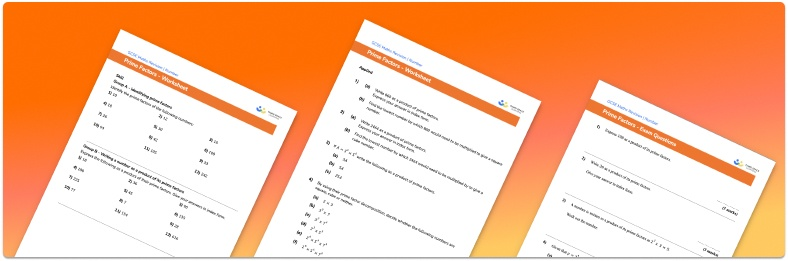# Prime Factors Worksheet• Section 1 of the prime factors  worksheet contains 20+ skills-based prime factors questions, in 3 groups to support differentiation
• Section 2 contains 3 applied prime factors questions with a mix of worded problems and deeper problem solving questions
• Section 3 contains 3 foundation and higher level GCSE exam style prime factors questions
• Answer keys and a mark scheme for all prime factors questions are provided
• Questions follow variation theory with plenty of opportunities for students to work independently at their own level
• All questions created by fully qualified expert secondary maths teachers
• Suitable for GCSE maths revision for AQA, OCR and Edexcel exam boards

• This field is for validation purposes and should be left unchanged.

You can unsubscribe at any time (each email we send will contain an easy way to unsubscribe). To find out more about how we use your data, see our privacy policy.

### Prime factors at a glance

The prime factors of a number are factors of that number which are prime. Every composite number can be written as a product of its prime factors

The factors of a number are values which divide exactly into the number. For example, the factors of 12 are 1, 2, 3, 4, 6 and 12.

Prime numbers are integers whose only factors are themselves and 1. Examples of prime numbers are 2, 3, 5, 7 and 11.

To find the prime factors of a number, we can draw a prime factor tree, breaking down the number into pairs of factors until we achieve all prime numbers. The prime factorisation of a number is often written using exponents, in index form, for example 72=2^(3)*3^(2).

The prime factors of two or more given numbers can be used to find the HCF and LCM of those numbers.

Looking forward, students can then progress to additional number worksheets, for example a multiplying and dividing decimals worksheet or an order of operations worksheet.For more teaching and learning support on Number our GCSE maths lessons provide step by step support for all GCSE maths concepts.

## Do you have KS4 students who need more focused attention to succeed at GCSE?There will be students in your class who require individual attention to help them succeed in their maths GCSEs. In a class of 30, it’s not always easy to provide.

Help your students feel confident with exam-style questions and the strategies they’ll need to answer them correctly with our dedicated GCSE maths revision programme.

Lessons are selected to provide support where each student needs it most, and specially-trained GCSE maths tutors adapt the pitch and pace of each lesson. This ensures a personalised revision programme that raises grades and boosts confidence.

Find out more